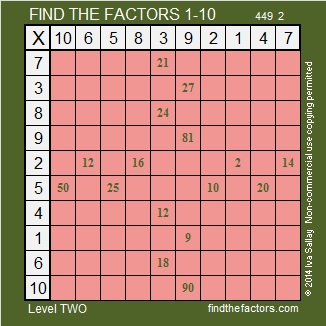# 319 and Level 2

• 319 is a composite number.
• Prime factorization: 319 = 11 x 29
• The exponents in the prime factorization are 1 and 1. Adding one to each and multiplying we get (1 + 1)(1 + 1) = 2 x 2 = 4. Therefore 319 has exactly 4 factors.
• Factors of 319: 1, 11, 29, 319
• Factor pairs: 319 = 1 x 319 or 11 x 29
• 319 has no square factors that allow its square root to be simplified. √319 ≈ 17.861Since 29 is one of its factors, 319 is the hypotenuse of a Pythagorean triple:

• 220-231-319 which is 11 times 20-21-29

This Level 2 puzzle has more than enough clues to help you find all of its factors:Print the puzzles or type the factors on this excel file: 10 Factors 2014-12-08